# Karnaugh Map

Boolean Algebra

In this tutorial we will learning about Karnaugh Map.

We know that truth table is used to represent values of a boolean function. Similarly, Karnaugh map is another way of representing the values of a boolean function. So, K-map is a table consisting of cells which represents a Minterm or Maxterm. Karnaugh map or K-map is named after Maurice Karnaugh.

## Karnaugh Map for Sum of Products

For SOP or Sum of Products, each cells in a K-map represents a Minterm. If there are n variables for a given boolean function then, the K-map will have 2n cells. And we fill the cells with 1s whose Minterms output is 1. Lets check the K-map for 2, 3 and 4 variables.

## 2 variables K-map for Sum of Products

Say we have two variables X and Y then, there will be 22 = 4 cells in the K-map.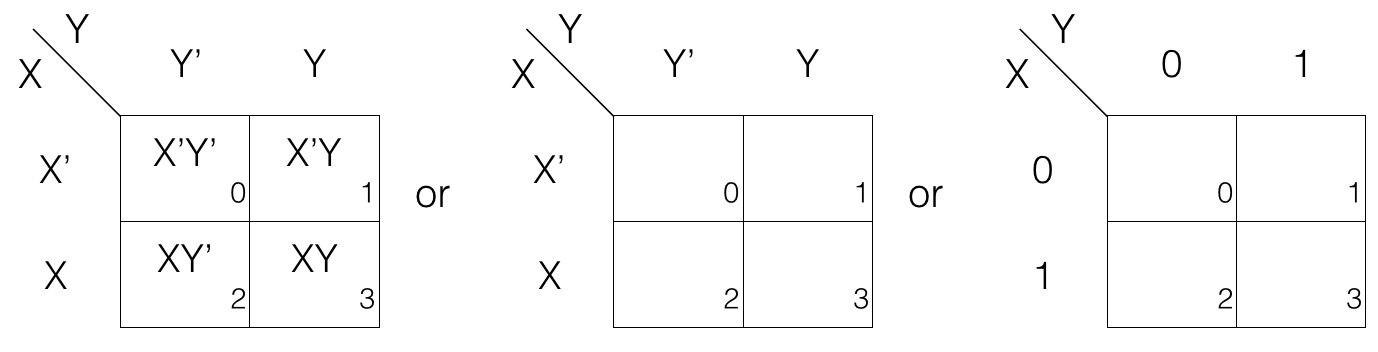Each cell has a subscripted number at the bottom right corner. It is the value of the minterm. So, if a cell has number 2 then it represent minterm m2.

## 3 variables K-map for Sum of Products

Say we have three variables X, Y and Z then there will be 23 = 8 cells in the K-map.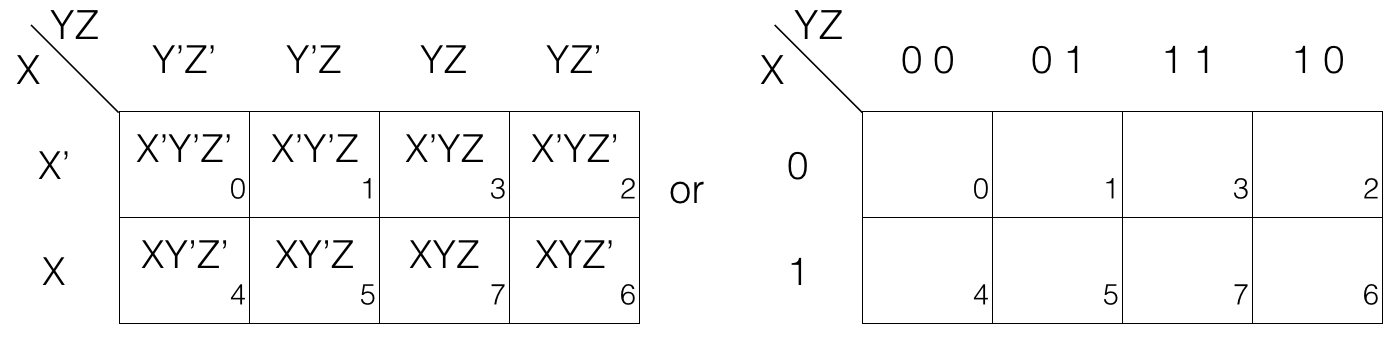Each cell has a subscripted number at the bottom right corner it is the value of the minterm. So, if a cell has number 7 then it represent minterm m7.

Now if we look at the above K-map we will see that the numbering scheme is 0, 1, 3, 2 in the first row and 4, 5, 7, 6 in the second row. So, the numbers differ in one place when moving from left to right.

00, 01, 11, 10 this is done so that only one variable change at a time from complement to un-complement or un-complement to complement every row.

Example, in the first row we have X’Y’Z’, X’Y’Z, X’YZ and X’YZ’ so, only one variable change at a time as we move from left to right.

1st row: moving left to right cell-wise
X’Y’Z’ —> X’Y’Z [Z’ changed to Z]
X’Y’Z —> X’YZ [Y’ changed to Y]
X’YZ —> X’YZ’ [Z changed to Z’]

## 4 variables K-map for Sum of Products

Say we have four variables W, X, Y and Z then there will be 24 = 16 cells in the K-map.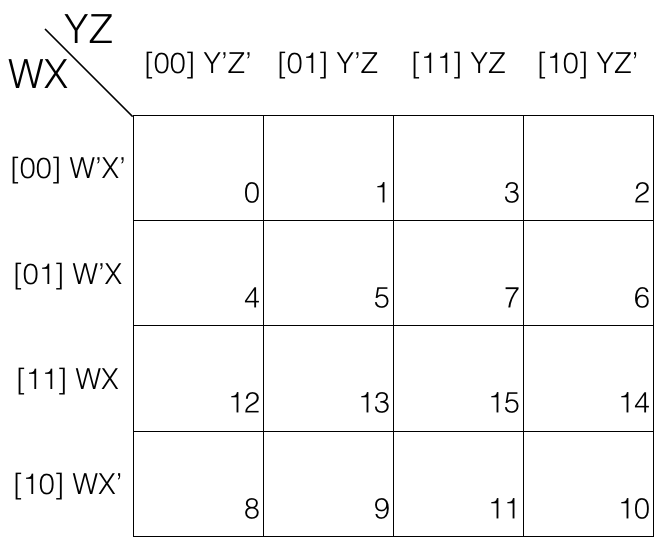Each cell has a subscripted number at the bottom right corner. It is the value of the minterm. So, if a cell has number 12 then, it represent minterm m12.

And in this case the numbering scheme is 0, 1, 3, 2 in the first row 4, 5, 7, 6 in the second row 12, 13, 15, 14 in the third row and 8, 9, 11, 10 in the fourth row.

## How to fill values in the K-map for Sum of Products?

Say we have a boolean function F defined on two variables A and B and F = 1 i.e., output is 1 only when exactly one of the input is 1. So, we can draw the following truth table with all possible input and output values.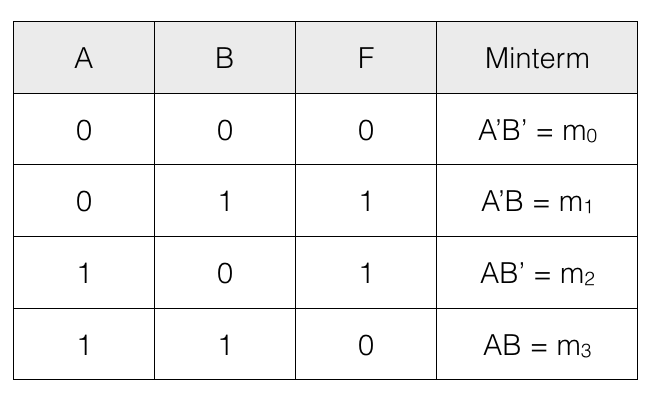To express F using Minterm shorthand notation we have to consider only those minterms for which F = 1.

F = m1 + m2

This can also be written as
F = ∑(1, 2)

Now, we have F = m1 + m2 = ∑(1, 2)
Draw an empty K-map having 4 cells (since there are 2 variables so 22 = 4 cells).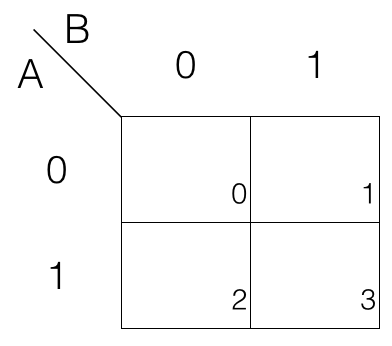F = m1 + m2
So, fill the cells marked with subscript 1 and 2 with value 1. And fill rest of the cells of the K-map with value 0.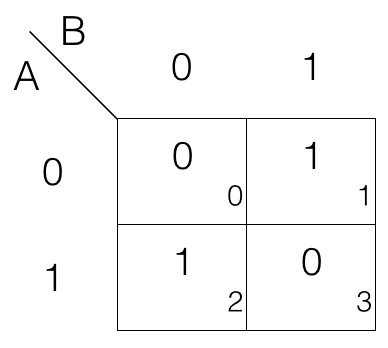In a similarly way we can fill 3 and 4 variables K-map.

## Karnaugh Map for Product of Sums

For POS each cells in a K-map represents a Maxterm. If there are n variables for a given boolean function then the K-map will have 2n cells. And we fill the cells with 0s whose Maxterm output is 0. Lets check the K-map for 2, 3 and 4 variables.

## 2 variables K-map for Product of Sums

Say we have two variables X and Y then there will be 22 = 4 cells in the K-map.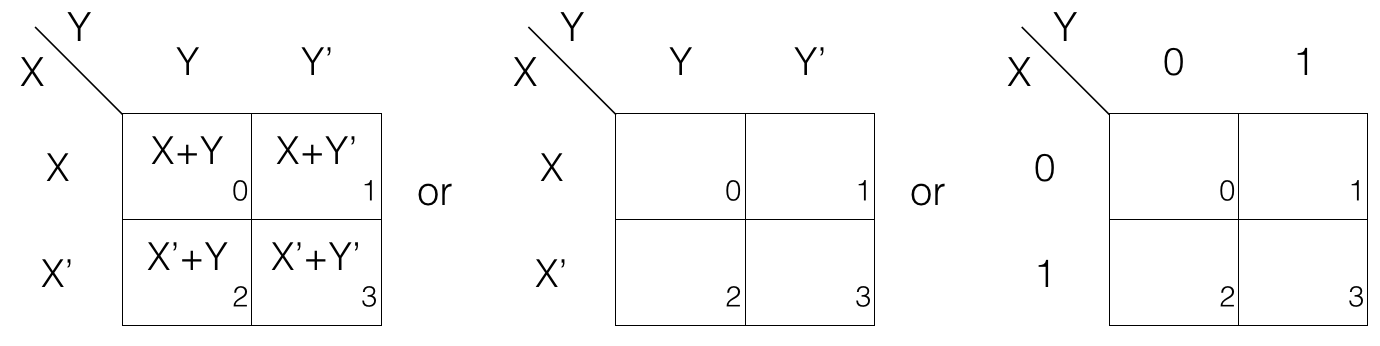Each cell has a subscripted number at the bottom right corner. It is the value of the maxterm. So, if a cell has number 2 then, it represent maxterm M2.

## 3 variables K-map for Product of Sums

Say we have three variables X, Y and Z then there will be 23 = 8 cells in the K-map. So, if a cell has number 0 then it represent maxterm M0.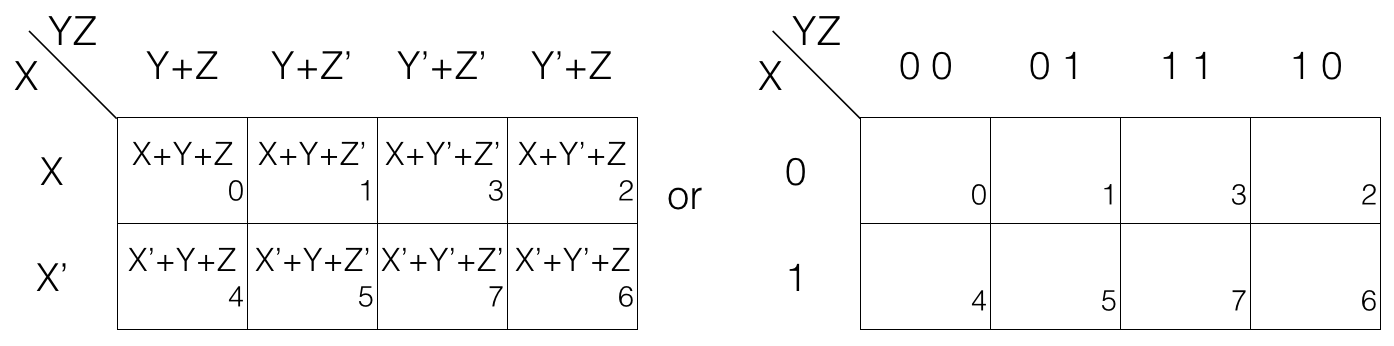Now if we look at the above K-map we will see that the numbering scheme is 0, 1, 3, 2 in the first row and 4, 5, 7, 6 in the second row. So, the numbers differ in one place when moving from left to right.

00, 01, 11, 10 this is done so that only one variable change at a time from complement to un-complement or un-complement to complement every row.

Example, in the first row we have X+Y+Z, X+Y+Z’, X+Y’+Z’ and X+Y’+Z so, only one variable change at a time as we move from left to right.

1st row: moving left to right cell-wise
X+Y+Z —> X+Y+Z’ [Z changed to Z’]
X+Y+Z’ —> X+Y’+Z’ [Y changed to Y’]
X+Y’+Z’ —> X+Y’+Z [Z’ changed to Z]

## 4 variables K-map for Product of Sums

Say we have four variables W, X, Y and Z then there will be 24 = 16 cells in the K-map.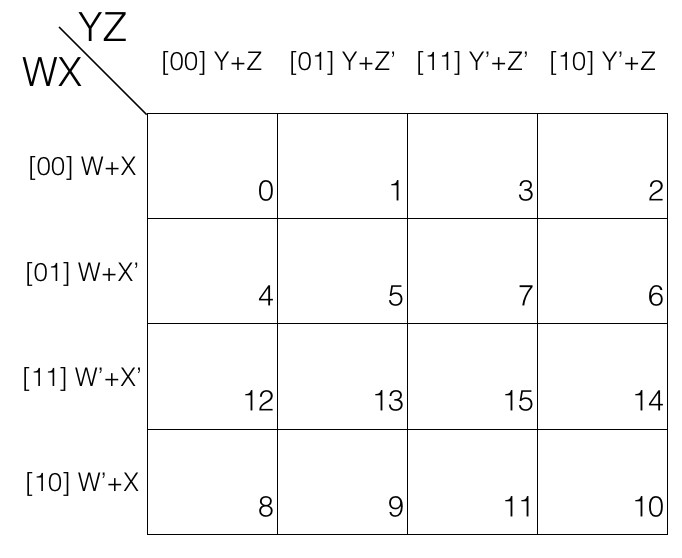Each cell has a subscripted number at the bottom right corner. It is the value of the maxterm. So, if a cell has number 15 then it represent maxterm M15.

And in this case the numbering scheme is 0, 1, 3, 2 in the first row 4, 5, 7, 6 in the second row 12, 13, 15, 14 in the third row and 8, 9, 11, 10 in the fourth row.

## How to fill values in the K-map for Product of Sums?

Say we have a boolean function F defined on two variables A and B and F = 1 i.e., output is 1 only when exactly one of the input is 1. So we can draw the following truth table with all possible input and output values.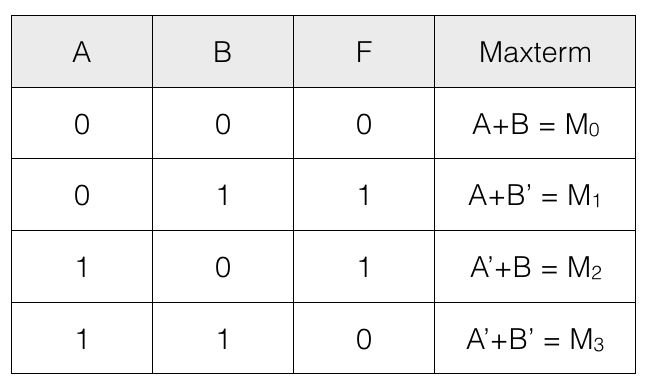To express F using Maxterm shorthand notation we have to consider only those maxterms for which F = 0.

F = M0 . M3

This can also be written as
F = ∏(0, 3)

Now that we have F = M0 . M3 = ∏(0, 3).

Draw an empty K-map having 4 cells (since there are 2 variables so 22 = 4 cells)F = M0 . M3
So, fill the cells marked with subscript 0 and 3 with value 0. And fill rest of the cells of the K-map with value 1.In a similarly way we can fill 3 and 4 variables K-map.